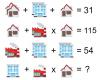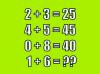BRAIN TEASERSBrain Teasers User Profile

# Tony Midas

rank
604
points
2
See full ranking list
short ranking list
 602 Sheden Mohammed 2 603 fatimah aram 2 604 Tony Midas 2 605 Adel Tarek 2 606 Sejal More 2
 Can you replace the question mark with a number? MATH PUZZLE: Can you replace the question mark with a number?Mathematical Puzzle: IF 2+3=... Mathematical Puzzle: IF 2+3=25, 4+5=45 and 0+8=40 THEN 1+6=?